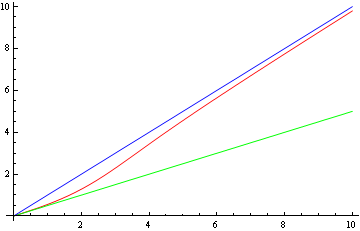# Robust prior illustration

In Bayesian statistics, prior distributions “get out of the way” as data accumulate. But some prior distributions get out of the way faster than others. The influence of robust priors decays faster when the data conflict with the prior.

Consider a single sample y from a Normal(θ, σ2) distribution. We consider two priors on θ, a conjugate Normal(0, τ2) prior and a robust Cauchy(0, 1) prior. We will look at the posterior mean of θ under each prior as y increases.

With the normal prior, the posterior mean value of θ is

y τ2/( τ2 + σ2).

That is, the posterior mean is always a fixed fraction of y. If the prior variance τ2 is large relative to the variance σ2 of the sampling distribution, the fraction will be closer to 1, but it will always be less than 1.

With the Cauchy prior, the posterior mean is

y – O(1/y)

as y increases. (See these notes if you’re unfamiliar with “big-O” notation.) So the larger y becomes, the closer the posterior mean of θ comes to the value of the data y.In the graph, the green line on bottom plots the posterior mean of θ with a normal prior as a function of y . The blue line on top is y. The red line in the middle is posterior mean of θ with a Cauchy prior. Note how the red line starts out close to the green line. That is, for small values of y, the posterior mean is nearly the same under the normal and Cauchy priors. But as y increases, red line approaches the blue line. The Cauchy prior has less influence as y increases.

In this graph σ = τ = 1. The results would be qualitatively the same for any values of σ and θ. If τ were larger relative to σ, the bottom line would be steeper, but the middle curve would still asymptotically approach the top line.

You can also show that with multiple samples, the posterior mean of θ converges more quickly to the empirical mean of the data when using a Cauchy prior than when using a normal prior if the mean is sufficiently large.

Update: See Asymptotic results for Normal-Cauchy model for proofs of the claims in this post.

Related post: Robust priors